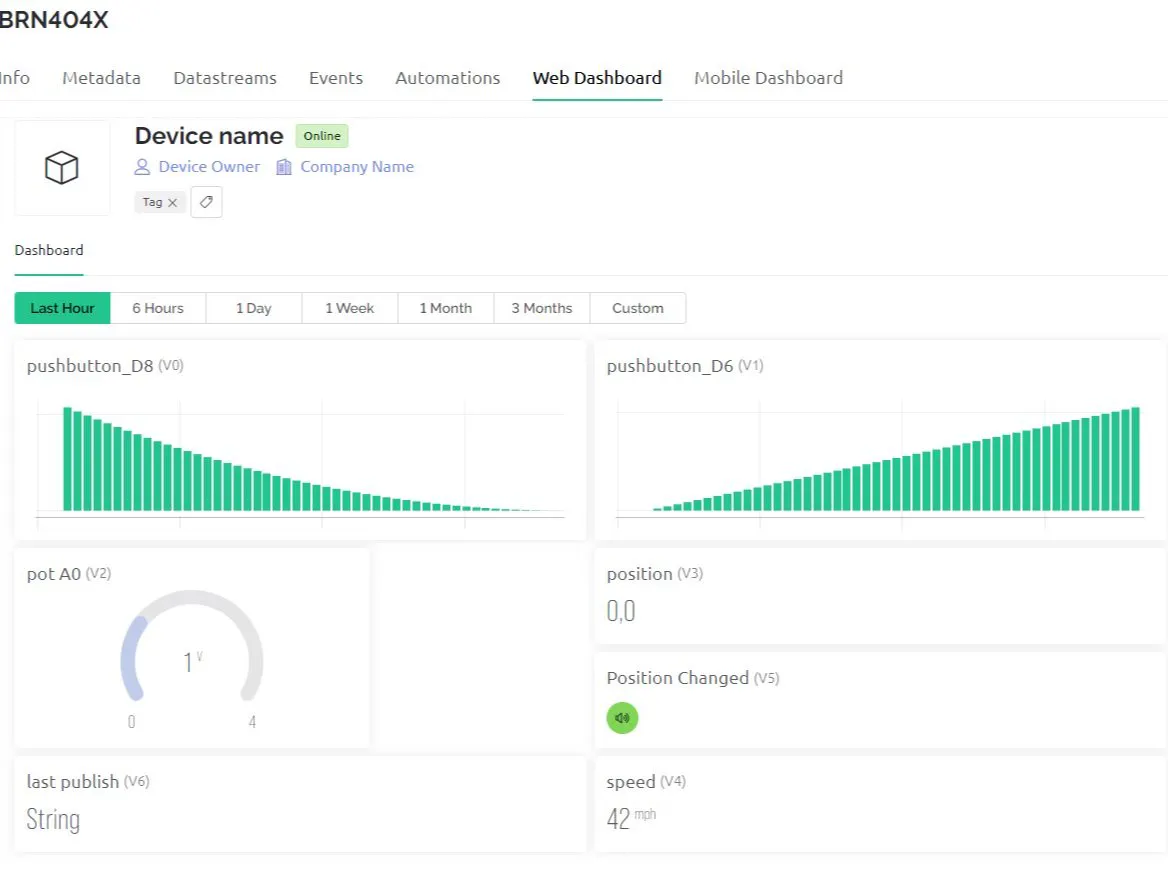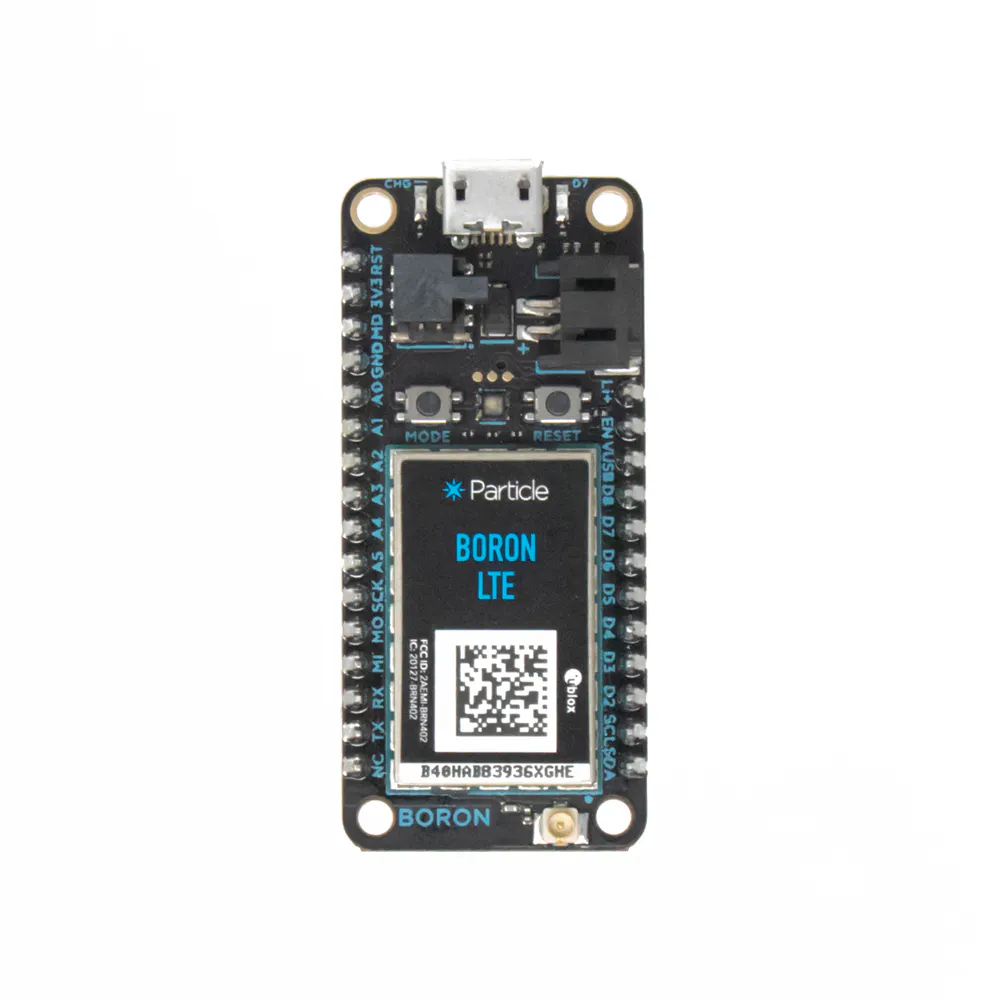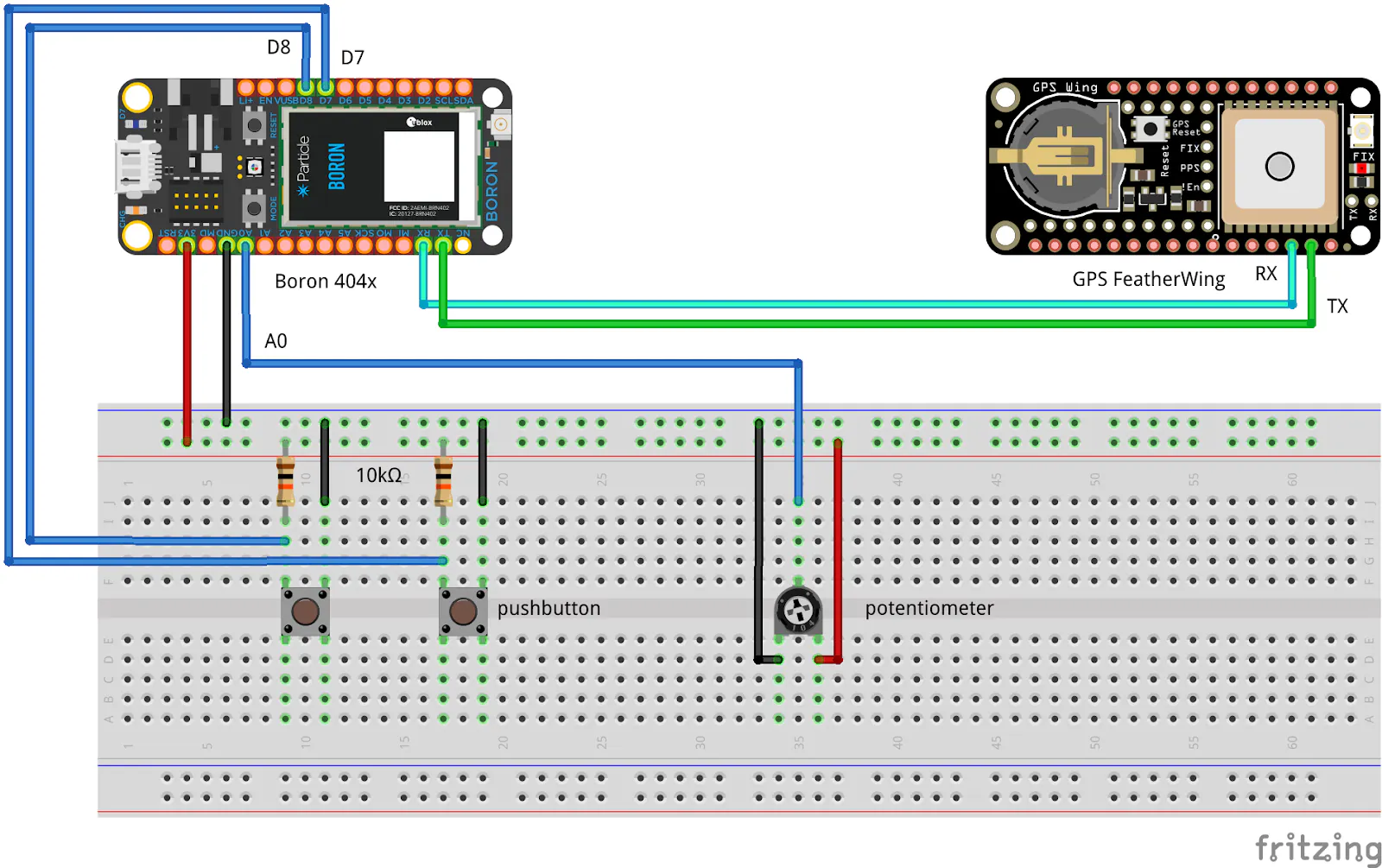# How to Connect a Particle Boron to Blynk IoT

How to push data from a Particle cellular device to the Blynk IoT platform.

IntermediateProtip15 hours5## Things used in this project

### Hardware componentsParticle Boron
×1

### Software apps and online servicesBlynk

## Schematics

### Fritzing sketch

Two pushbuttons and a potentiometer as inputs to the Particle Boron.## Code

### Particle sketch for Boron that pushes data to Blynk via a Particle webhook.

C/C++
Firmware for Boron
```/*
Project particle_to_blynk.io
Author: Mark Kiehl / Mechatronic Solutions LLC
Date: March 2023

Publish to Blynk via the Particle webhook only if either of the two
digital inputs has changed state, or if the device position has changed
by more than 122 m or 400 ft.

Built-in blue LED on D7:
Turns on constant during setup, then breathes when called in loop() unless:
mode 4 (fast burst every 1 s) if GPS cannot get a fix.
mode 3 (slow burst every 1 second) when data publishing is pending.

The GPS red LED blinks at about 1Hz while it's searching for satellites,
and blinks once every 15 seconds when a fix is found.

Hardware:
Particle Boron 404x

Software:
Custom code for sending data to Particle Webhook for Blynk.

*/

#include "Particle.h"

const char* firmware_version = "0.0.0";
uint8_t led_mode = 0;
boolean just_started = true;
#define BLYNK_AUTH_TOKEN "fhG3U-TZNwezrLb1FMdSGzS23WvdlpIQ"

/////////////////////////////////////////////////////////////////////////
uint8_t LEDlastMode = 0;

void blinkLEDnoDelay(byte pin, byte mode) {
// Blink the LED on 'pin' without using delay() according to
// the 'mode' argument defined below.
// pin must support PWM.
//
// mode:
// 0 = breathing
// 1 = blink slow constantly
// 2 = blink fast constantly
// 3 = slow burst every 1 second
// 4 = fast burst every 1 second
//
if (mode == 0) {
// breathing
if (LEDlastMode != mode) {
digitalWrite(pin, LOW);
}
} else {
}
LEDlastMode = mode;
}
} else if (mode == 1) {
LEDlastMode = mode;
}
} else if (mode == 2) {
LEDlastMode = mode;
}
} else if (mode == 3) {
// slow burst every 1 second
// Slow 4 blinks (lazy burst) followed by 1 sec pause
if (LEDlastMode != mode) {
}
} else {
digitalWrite(pin, LOW);
}
LEDlastMode = mode;
}
} else if (mode == 4) {
// fast burst every 1 second
// Fast 4 blinks (burst) followed by 1 sec pause
if (LEDlastMode != mode) {
}
} else {
digitalWrite(pin, LOW);
}
LEDlastMode = mode;
}
} // mode

// S-O-S
const uint8_t S = 150;
const uint16_t O = 300;
for(uint8_t i = 3; i>0; i--){
digitalWrite(ledPIN, HIGH);
delay(S);
digitalWrite(ledPIN, LOW);
delay(S);
}
delay(200);
for(uint8_t i = 3; i>0; i--){
digitalWrite(ledPIN, HIGH);
delay(O);
digitalWrite(ledPIN, LOW);
delay(O);
}
delay(200);
for(uint8_t i = 3; i>0; i--){
digitalWrite(ledPIN, HIGH);
delay(S);
digitalWrite(ledPIN, LOW);
delay(S);
}
delay(200);

/////////////////////////////////////////////////////////////////////////
// digital inputs

// Bundle all of the digital input data into a structure.
const uint8_t DI_COUNT = 2;
// initialize DI_DEFAULT_STATE LOW if pulldown resistor, HIGH if pullup resistor.
// Must use the same LOW / HIGH (pullup / pulldown) for all digital inputs monitored.
// timer_interval and timer_last are used for debounce.
const uint8_t DI_DEFAULT_STATE = HIGH;
struct digital_inputs_t {
uint8_t pin;
uint8_t state;
uint8_t last_state;
uint32_t timer_interval;
uint32_t timer_last;
boolean alarm;
uint32_t state_change_count;
};
digital_inputs_t arr_di[DI_COUNT];

void ProcessDigitalInputs() {
// Publish the arr_di[i].state_change_count ONLY when the change in state is
// from DI_DEFAULT_STATE to !DI_DEFAULT_STATE.
// Look for a change in state (HIGH/LOW) for the digital inputs referenced by arr_di.
// If the change in state is from DI_DEFAULT_STATE to !DI_DEFAULT_STATE, and
// arr_di[i].alarm == false, then increment arr_di[i].state_change_count and
// publish the change (arr_di[i].alarm = true).
for (uint8_t i=0; i<DI_COUNT; i++) {
if (arr_di[i].timer_last > millis()) arr_di[i].timer_last = millis();
if (arr_di[i].state != arr_di[i].last_state && millis() - arr_di[i].timer_last > arr_di[i].timer_interval) {
// Change in state for arr_di[i].pin detected.
if (arr_di[i].state != DI_DEFAULT_STATE && arr_di[i].alarm == false) {
led_mode = 3; // 3 = slow burst every 1 second
arr_di[i].state_change_count++; // Only count the change from DI_DEFAULT_STATE to !DI_DEFAULT_STATE. (HIGH = bilge switch off) to LOW = bilge switch on).
arr_di[i].alarm = true; // Causes the arr_di[i].state_change_count to be published by publishTimer()
}
arr_di[i].last_state = arr_di[i].state;
arr_di[i].timer_last = millis();
}
} // for
} // ProcessDigitalInputs()

////////////////////////////////////////////////////////////////
// Install library "Adafruit_GPS" from the Particle cloud.
// Serial1 is also UART1 on the Boron/Argon/Xenon pins D10/Rx and D9/Tx
#define GPSSerial Serial1
// Connect to the GPS on the hardware port
// Set GPSECHO to 'false' to turn off echoing the GPS data to the Serial console
// Set to 'true' if you want to debug and listen to the raw GPS sentences
#define GPSECHO false

/////////////////////////////////////////////////////////////////////////
// Particle publish to webhook / Blynk only when something happens.

const uint32_t TIMER_INTERVAL_MS = 60000;  // Used to limit the frequency of publishing. Use 5 min or 300000 ms
uint32_t last_publish_ms = 0;
float lat = 0.0;
float lon = 0.0;
float mph = 0.0;
float vdc_batt = 0.0;
bool publish_to_blynk = false;
uint8_t loc = 0;  // 1 when the GPS position of the device has changed by more than 122 m or 400 ft.

void publishTimer() {
if (last_publish_ms > millis()) last_publish_ms = millis();
if (millis() - last_publish_ms >= TIMER_INTERVAL_MS) {

// Publish to Blynk if any arr_di[i].alarm == true, on first restart,
// or if the device's position has changed by more than 122 m or 400 ft.
publish_to_blynk = false;

// Determine if any DI has alarm = true
for (int i=0; i<DI_COUNT; i++) {
if (arr_di[i].alarm == true) {
publish_to_blynk = true;
}
} // for

uint32_t v0 = arr_di.state_change_count;
uint32_t v1 = arr_di.state_change_count;
float pot = 0.0; // V2
//uint32_t V2 = arr_di.state_change_count;
//uint32_t V3 = arr_di.state_change_count;
loc = 0; // location has not changed (default).

if (GPS.fix) {
Serial.printlnf("GPS UTC %4d-%02d-%02dT%02d:%02d:%02d%+05d, fix qual %d, sat %d, lat %0.5f, lon %0.5f, mph %0.1f, %0.1f deg, altitude %0.1f m, mag var %0.1f deg", GPS.year+2000, GPS.month, GPS.day, GPS.hour, GPS.minute, GPS.seconds, 0, GPS.fixquality, GPS.satellites, GPS.latitudeDegrees, GPS.longitudeDegrees, GPS.speed, GPS.altitude, GPS.angle, GPS.magvariation);
if ((int)lat == 0 || (int)lon == 0) {
lat = GPS.latitudeDegrees;
lon = GPS.longitudeDegrees;
mph = GPS.speed * 1.15078; // convert the speed in knots to mph
}

// Simulate location change
//lat = lat + 0.0012; // more than 400 ft
//Serial.printlnf("Latitude/Longitude: %f, %f", lat, lon);

// FIX: 0 means no 'valid fix', 1 means 'normal precision', and 2 means the position data is further corrected by some differential system. Typically 1 with internal antenna, 2 with external antenna.
// Sat: => 4 typical, but over time in home varies from 4 to 6 with internal antenna. With external antenna, typically 8 to 10.
// Note that knots will float at 0.1 to 1.2 when the GPS is at rest with the internal antenna. With external antenna, value is 0.0 to 0.01 knots.
// Lat/Lon in decimal degrees to 3 decimal places is 111 m / 364 ft, to 4 places = 11.1 m or 36.4 ft.
// A change in GPS latitude/latitude of 0.0011 is a distance of 122 m or 400 ft.
//if (GPS.fixquality > 0 && (fabs(GPS.latitudeDegrees - lat) > 0.001 || fabs(GPS.longitudeDegrees - lat) > 0.001)) {
if (GPS.fixquality > 0 && (fabs(fabs(GPS.latitudeDegrees) - fabs(lat)) > 0.0011 || fabs(fabs(GPS.longitudeDegrees) - fabs(lon)) > 0.0011)) {
double delta_lat_m = fabs(fabs(GPS.latitudeDegrees) - fabs(lat))*10000.0/90.0*1000.0; // distance in m
double delta_lon_m = fabs(fabs(GPS.longitudeDegrees) - fabs(lon))*10000.0/90.0*1000.0;
double delta_lat_ft = fabs(fabs(GPS.latitudeDegrees) - fabs(lat))*10000.0/90.0*3280.4; // distance in ft
double delta_lon_ft = fabs(fabs(GPS.longitudeDegrees) - fabs(lon))*10000.0/90.0*3280.4;
double delta_m = max(delta_lat_m, delta_lon_m);
double delta_ft = max(delta_lat_ft, delta_lon_ft);
publish_to_blynk = true;
loc = 1;
Serial.printlnf("The device has moved a distance of %f m or %f ft since the last time the GPS position was reported", delta_m, delta_ft);
}
if (GPS.fixquality > 0 && just_started == true) {
publish_to_blynk = true;
just_started = false;
}
lat = GPS.latitudeDegrees;
lon = GPS.longitudeDegrees;
mph = GPS.speed * 1.15078; // convert the speed in knots to mph
if (led_mode != 3) led_mode = 0;
} else {
led_mode = 4; // 4 = fast burst every 1 second because no GPS fix.
Serial.println("NO GPS fix!");
} // GPS.Fix

if (publish_to_blynk == true) {
char data; // See serial output for the actual size in bytes.

// 12 bit ADC (values between 0 and 4095 or 2^12) or a resolution of 0.8 mV

// Note the escaped double quotes around the value for BLYNK_AUTH_TOKEN.
snprintf(data, sizeof(data), "{\"t\":\"%s\",\"v0\":%lu,\"v1\":%lu,\"pot\":%.1f,\"lat\":%f,\"lon\":%f,\"spd\":%f,\"moved\":%u}", BLYNK_AUTH_TOKEN, v0, v1, pot, lat, lon, mph, loc);
//snprintf(data, sizeof(data), "{\"t\":%s,\"v0\":%lu,\"v1\":%lu,\"pot\":%.1f,\"lat\":%f,\"lon\":%f,\"spd\":%f,\"moved\":%u}", BLYNK_AUTH_TOKEN, v0, v1, pot, lat, lon, mph, loc);
Serial.printlnf("Sending to Blynk: '%s' with size of %u bytes", data, strlen(data));
bool pub_result = Particle.publish("blynk_https_get", data, PRIVATE);
if (pub_result) {
for (int i=0; i<DI_COUNT; i++) {
arr_di[i].alarm = false;
}
publish_to_blynk = false;
if (led_mode != 4) led_mode = 0;
} else {
Serial.println("ERROR: Particle.publish()");
}
last_publish_ms = millis(); // Limit publish frequency to limit cellular data usage.
} else {
last_publish_ms = millis() - 2000; // Don't check if publish required too frequently.
} // publish_to_blynk

}
} // publishTimer()

void setup() {

pinMode(D7, OUTPUT);
digitalWrite(D7, HIGH);

Serial.begin(9600);
waitFor(Serial.isConnected, 30000);
delay(1000);
Serial.printlnf("Device OS v%s", System.version().c_str());
Serial.printlnf("Free RAM %lu bytes", System.freeMemory());
Serial.printlnf("Firmware version v%s", firmware_version);

// Note: If the GPS FeatherWing is not attached, the code continues.
GPS.begin(9600);
// Turn on RMC (recommended minimum) and GGA (fix data) including altitude
GPS.sendCommand(PMTK_SET_NMEA_OUTPUT_RMCGGA);
GPS.sendCommand(PMTK_SET_NMEA_UPDATE_1HZ); // Set 1 Hz update rate
delay(1000);

// Initialize arr_di
arr_di.pin = D8;
arr_di.pin = D6;
for (uint8_t i=0; i<DI_COUNT; i++) {
if (DI_DEFAULT_STATE == LOW) {
pinMode(arr_di[i].pin, INPUT);
arr_di[i].state = LOW;
arr_di[i].last_state = LOW;
} else {
pinMode(arr_di[i].pin, INPUT);
arr_di[i].state = HIGH;
arr_di[i].last_state = HIGH;
}
arr_di[i].timer_interval = 50; // debounce timer
arr_di[i].timer_last = millis();
arr_di[i].alarm = false;
arr_di[i].state_change_count = 0;
}

pinMode(A0, INPUT);

delay(5000); // Give the Boron and GPS time to connnect.

randomSeed(millis());
digitalWrite(D7, LOW);
Serial.println("Setup complete");
} // setup()

void loop() {

ProcessDigitalInputs();

// Below absolutely required here.
if (GPSSerial.available()) {
if (GPS.parse(GPS.lastNMEA())) {
if (!GPS.parse(GPS.lastNMEA())) {
// sets the newNMEAreceived() flag to false
}
}
} // GPS
}

publishTimer();